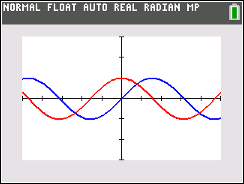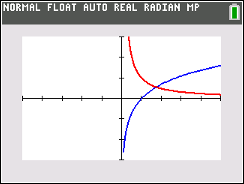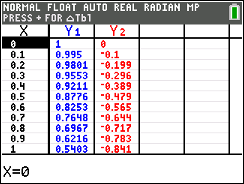••• ##### Device
• TI-83 Plus Family
• TI-84 Plus
• TI-84 Plus Silver Edition
•TI-84 Plus C Silver Edition
•TI-84 Plus CE

# Calculus: Investigating the Derivatives of Some Common Functions

by Texas Instruments#### Overview

In this activity, students will investigate the derivatives of sine, cosine, natural log, and natural exponential functions by examining the symmetric difference quotient at many points. They develop the idea of the derivative as a function. They gather evidence toward some common derivative formulas. They also use numerical and graphical investigations to form conjectures.

#### Key Steps

•Students will develop the idea of the derivative as a function.

•Students will gather evidence toward some common derivative formulas.

•Students will use numerical and graphical investigations to form conjectures.